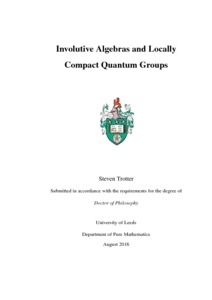# Involutive Algebras and Locally Compact Quantum Groups

Trotter, Steven (2016) Involutive Algebras and Locally Compact Quantum Groups. PhD thesis, University of Leeds.Preview
Text
Trotter_S_Mathematics_PhD_2016.pdf - Final eThesis - complete (pdf)
Available under License Creative Commons Attribution-Noncommercial-Share Alike 2.0 UK: England & Wales.

Download (1985Kb) | Preview

## Abstract

In this thesis we will be concerned with some questions regarding involutions on dual and predual spaces of certain algebras arising from locally compact quantum groups. In particular we have the $L^1(\G)$ predual of a von Neumann algebraic quantum group $(L^\infty(\G), \Delta)$. This is a Banach algebra (where the product is given by the pre-adjoint of the coproduct $\Delta$), however in general we cannot make this into a Banach $*$-algebra in such a way that the regular representation is a $*$-homomorphism. We can however find a dense $*$-subalgebra $L^1_\sharp(\G)$ that satisfies this property and is a Banach algebra under a new norm. This was originally considered by Kustermans and Vaes when defining the universal C$^*$-algebraic quantum group, however little else has been studied regarding this algebra in general. In this thesis we study the $L^1_\sharp$-algebra of a locally compact quantum group in this thesis. In particular we show how this has a (not necessarily unique) operator space structure such that this forms a completely contractive Banach algebra, we study some properties for compact quantum groups, we study the object for the compact quantum group $\mathrm{SU}_q(2)$ and we study the operator biprojectivity of the $L^1_\sharp$-algebra. In addition we also briefly study some related properties of $C_0(\G)^*$ and its $*$-subalgebra ${C_0(\G)^*}_\sharp$.

Item Type: Thesis (PhD) Locally Compact Quantum Groups Banach Algebras C*-algebras The University of Leeds > Faculty of Maths and Physical Sciences (Leeds) > School of Mathematics (Leeds) > Pure Mathematics (Leeds) uk.bl.ethos.703348 Dr Steven Trotter 20 Feb 2017 11:47 25 Jul 2018 09:54 http://etheses.whiterose.ac.uk/id/eprint/16168

You do not need to contact us to get a copy of this thesis. Please use the 'Download' link(s) above to get a copy.
You can contact us about this thesis. If you need to make a general enquiry, please see the Contact us page.